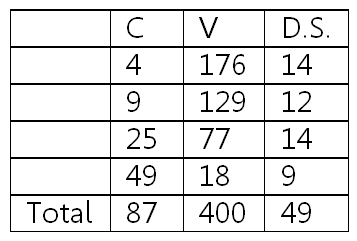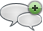### Chapters Whose Number is the Square of a Prime Number

#### الخميس، 23 إبريل 2015 - 1:14There are four chapters in the Quran that have chapter numbers that are squares of primes; namely chapters 4 (2^2), 9 (3^2), 25 (5^2) and 49 (7^2). These chapters exhibit interesting mathematical relationships between their Chapter number, C, their number of verses, V, and the digit sum of the number of verses, D.S. (the sum of the digits that make up the number; e.g. 176 had a digit sum of 1+7+6 = 14):

 D.S. V C 14 176 4 12 129 9 14 77 25 9 18 49 49 400 87 Total

RESULTS

The total number of verses is 400 which is a perfect square: 400 = 20*20.

The total of the digit sums of the chapter numbers is a perfect square: 49 = 7*7.

Digit sum of the total of the number of verses is 4+0+0 = 4 which is a perfect square = 2*2.

The sum of the total number of verses + the total of the chapter numbers is 400+87 = 487 which is additive prime (a prime number whose digit sum, 4+8+7=19, is also a prime).

The sum of the total number of verses + the total of the digit sum of the number of verses is 400+49 = 449 which is also additive prime (its D.S. is 4+4+9=17).

The concatenation of the total number of verses and the total of the chapter numbers, 40087 is also additive prime.أضف تعليقك
##### رجاء ضرورة الإلتزام بعدم إضافة أي تعليق يمس أو يسيء للأديان أو المعتقدات أو المقدسات, كما نرجو ألا يتضمن التعليق السباب أو أي ألفاظ تخدش الحياء والذوق العامتعليقات Chapters whose number is the square of a composite number. تعليق: Ali Adams الخميس، 23 إبريل 2015 - 5:25 Interestingly, these chapters have a total number of ayas that is an additive prime.

Chapter 16 has 128 ayas, chapter 36 has 83 ayas, chapter 81 has 18 ayas and chapter 100 has 11 ayas for a total of 269. This is a prime number. The sum of its digits is 17 which is also prime, making the number an additive prime..
 الأكثر قراءة333667.الحديد.الكهف.السور التي يتدأ بــ "يَٰٓأَيُّهَا ٱلَّذِينَ ءَامَنُوٓا۟ " مجموعها 114.فبهت الذي كفر.ذكر موسى عليه السلام في القرآن.مزيد من الاثبات ان عاد الثانية هي امريكا.Number 114 is an Indication of Completeness.Sura Alfati7a, Alfalaq and Annas all use 21 Letters of the Alphabet.ملاحظة في ترتيب سور فصلت والشورى وق.
الموقع الرسمي حم © 2015, كل الحقوق محفوظة |